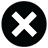# 13 Question MethodChuck Berry Lyrics

The 'thirteen question method' (Chuck Berry)

The 'thirteen question method' is the one to use
The 'thirteen question method' is the one to use
The 'thirteen question method' is the one
To use when you want to go have some fun
The 'thirteen question method' is the one to useQuestion number one: let's have some fun
Question number two is: what to do ?
Question number three is: will you dine and dance with me?
Question number four: out the door?

Question number five: I want you to know jive
Question number six: how long to get fixed ?
Question number seven: should I pick you up at a quarter to eleven?
Question number eight: is it a date ?

(guitar solo)

Question number nine is: where to dine ?
Question number ten: can we get in?
Question number eleven: it'll be just like heaven
Question number twelve when we're by ourselves

The 'thirteen question method' is the one to use
The 'thirteen question method' is the one to use
The 'thirteen question method' is the one
To use when you want to go have some fun
The 'thirteen question method' is the one to use

Lyrics © ENTERTAINMENT ONE U.S. LP
Written by: CHUCK BERRY

Lyrics Licensed & Provided by LyricFind
To comment on specific lyrics, highlight them